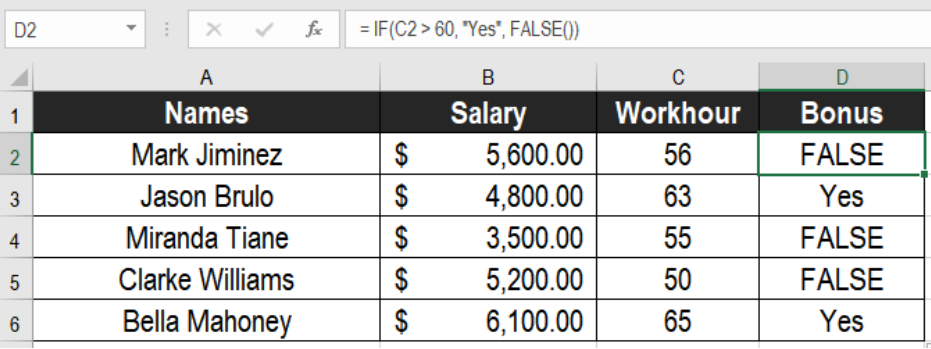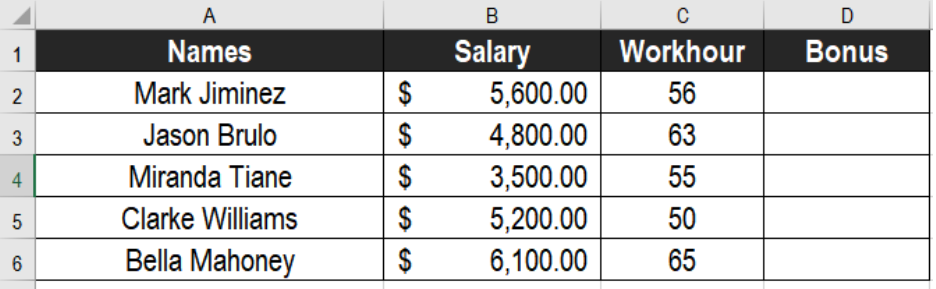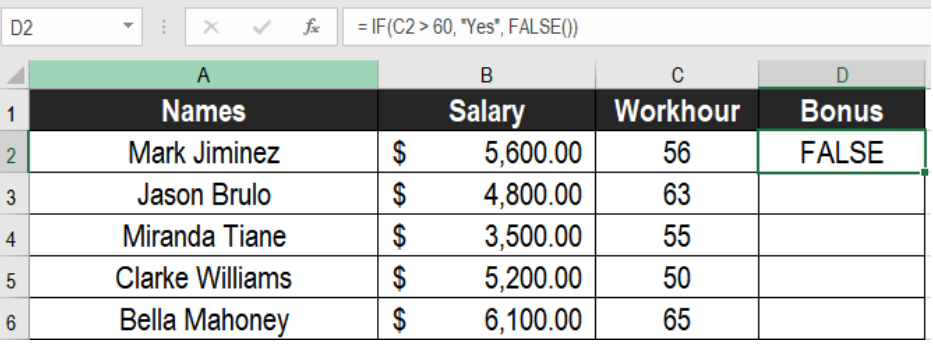Get instant live expert help with Excel or Google Sheets“My Excelchat expert helped me in less than 20 minutes, saving me what would have been 5 hours of work!”

#### Post your problem and you’ll get Expert help in seconds.

Your message must be at least 40 characters
Our professional Expert are available now. Your privacy is guaranteed.

# Excel FALSE Function

The FALSE function in Excel is a logical functions used in mathematical calculations. It provides a logical value while working on a worksheet. We can combine this function with the true function. Combining with other functions such as – IF function, it can be very useful. In this tutorial, we will learn how to use the FALSE function in Excel.Figure 1. Example of How to use the Excel FALSE Function

## Syntax

The general formula is very straightforward comparing to other functions of Excel. The syntax is:

`=FALSE()`

From the syntax, we can see that the FALSE function does not take any arguments. The Excel FALSE function is equivalent to the value FALSE directly. This formula returns the logical value FALSE based on a calculation. It does not return any other value than FALSE.

## Setting Up Data

The following example uses an employee database. Column A, B and C has the employee names, salary and work hours.Figure 2. The Sample Data Set

To find out if they qualify for bonuses in column D, we need to:

• Go to cell D2.
• Assign the Formula `= IF(C2 > 60, Yes, FALSE())`
• Press Enter.Figure 3. Applying the Formula to the Data Set

• Drag the formula from cells D2 to D6 to apply it to the entire column.

This will make column D show if the employees qualify for the bonuses. The Yes values in column D represent the employees that are eligible for a bonus.

## Note

The Excel FALSE function is labeled as a “compatibility function”. It is used to be compatible with other spreadsheet applications.

Most of the time, the problem you will need to solve will be more complex than a simple application of a formula or function. If you want to save hours of research and frustration, try our live Excelchat service! Our Excel Experts are available 24/7 to answer any Excel question you may have. We guarantee a connection within 30 seconds and a customized solution within 20 minutes.

### Did this post not answer your question? Get a solution from connecting with the expert.Another blog reader asked this question today on Excelchat:
Solution examplesGiven: RetYr = 2057 B48=2055 B49=2056 B50=2057 FirstYrAnnExp = 174,855 B51=2058 M48=IF(B48>=RetYr-1,IF(M47>1,M47*(1+Inflation),FirstYrAnnExp)) Returns FALSE M49=IF(B49>=RetYr-1,IF(M48>1,M48*(1+Inflation),FirstYrAnnExp)) Correctly retuns 0 M50=IF(B50>=RetYr-1,IF(M49>1,M49*(1+Inflation),FirstYrAnnExp)) Correctly returns 174,855 M51=IF(B51>=RetYr-1,IF(M50>1,M50*(1+Inflation),FirstYrAnnExp)) Correctly returns 180,100 How can I avoid â€œFALSEâ€ when the B column is less than RetYr? A zero or blank would be better.
Solved by V. W. in 20 minsHi Can you see any glaring error with the below formula and help me why it wont work? =IF(E8<400,J6,(IF(E8<=1200,J5)),(IF(E8>=1201,J4)))*E8 Thanks Em
Solved by V. C. in 40 minsI need a formula that looks up if the date (which looks like "8/1/2018 8:05:36 AM") in Column "C" contains year 2018. And then populates "2018" in Column D, if the date does not contain "2018" then enter False in Column D.
Solved by F. H. in 20 minsI am trying to have a tab change color when the Sum of a row = 0. I am using code on the tab but it appears to be confused because the value of the given cell is not 0 it is "=SUM(G4:G17)"
Solved by S. F. in 41 minsCould you help me by explaining the IF function?
Solved by G. L. in 24 mins## Subscribe to Excelchat.coAnother blog reader asked this question today on Excelchat: